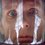# Playing with Integrals: Limits and Integrals 4

This note wasn't a planned one, but yesterday I tried to solve a very beautiful problem proposed to me by Gabriel Stuart Romon. The problem asked to find the following limit

$\lim_{n\to\infty}\int^b_a f(x)|\sin(nx)|\,dx, \forall f(x)\subset C^0 [a,b].$

The detailed solution to this problem, written by Gabriel may be found here. However, I want to discuss another problem, which I found on math.stackexchange. The key idea of it helped me to elaborate the solution to Gabriel's problem. I hope you'll find it beautiful and interesting.

Problem. If $g(x):\mathbb{R}\rightarrow\mathbb{R}$ is a continuous periodic function with period 1 and $f(x):[0,1]\rightarrow\mathbb{R}$ is a continuous function, prove that $\lim_{n\to\infty}\int^1_0 f(x)g(nx)\,dx=\left(\int^1_0 f(x)\,dx\right)\left(\int^1_0 g(x)\,dx\right).$

Solution. First of all we'll break our single integral into the sum of integrals over the shorter intervals.

$\int^1_0 f(x)g(nx)\,dx=\sum^{n-1}_{i=0}\int^{\frac{i+1}{n}}_{\frac{i}{n}} f(x)g(nx)\,dx=$ $=\frac{1}{n}\left(\sum^{n-1}_{i=0}\int^{i+1}_i f\left(\frac{u}{n}\right)g(u)\,du\right)$

By applying mean-value theorem we'll transform our sum into something similar to a Riemann sum and we'll use the fact that $g(x)$ is periodic in our favor.

$\frac{1}{n}\left(\sum^{n-1}_{i=0}\int^{i+1}_{i} f\left(\frac{u}{n}\right)g(u)\,du\right)=\frac{1}{n}\sum^{n-1}_{i=0}\left(f(\xi_i)\int^{i+1}_{i} g(u)\,du\right)=$ $=\frac{1}{n}\left(\sum^{n-1}_{i=0}f(\xi_i)\right)\left(\int^1_0 g(u)\,du\right)$

Because every $\xi_i\in\left[\dfrac{i}{n},\dfrac{i+1}{n}\right]$, we have that the limit of our sum is $\int^1_0 f(x)\, dx$, so

$\lim_{n\to\infty}\int^1_0 f(x)g(nx)\,dx=\lim_{n\to\infty}\left(\frac{1}{n}\sum^{n-1}_{i=0}f(\xi_i)\right)\left(\int^1_0 g(x)\,dx\right)=$ $=\left(\int^1_0 f(x)\,dx\right)\left(\int^1_0 g(x)\,dx\right).$Note by Nicolae Sapoval
6 years, 7 months ago

This discussion board is a place to discuss our Daily Challenges and the math and science related to those challenges. Explanations are more than just a solution — they should explain the steps and thinking strategies that you used to obtain the solution. Comments should further the discussion of math and science.

When posting on Brilliant:

• Use the emojis to react to an explanation, whether you're congratulating a job well done , or just really confused .
• Ask specific questions about the challenge or the steps in somebody's explanation. Well-posed questions can add a lot to the discussion, but posting "I don't understand!" doesn't help anyone.
• Try to contribute something new to the discussion, whether it is an extension, generalization or other idea related to the challenge.

MarkdownAppears as
*italics* or _italics_ italics
**bold** or __bold__ bold
- bulleted- list
• bulleted
• list
1. numbered2. list
1. numbered
2. list
Note: you must add a full line of space before and after lists for them to show up correctly
paragraph 1paragraph 2

paragraph 1

paragraph 2

[example link](https://brilliant.org)example link
> This is a quote
This is a quote
    # I indented these lines
# 4 spaces, and now they show
# up as a code block.

print "hello world"
# I indented these lines
# 4 spaces, and now they show
# up as a code block.

print "hello world"
MathAppears as
Remember to wrap math in $$ ... $$ or $ ... $ to ensure proper formatting.
2 \times 3 $2 \times 3$
2^{34} $2^{34}$
a_{i-1} $a_{i-1}$
\frac{2}{3} $\frac{2}{3}$
\sqrt{2} $\sqrt{2}$
\sum_{i=1}^3 $\sum_{i=1}^3$
\sin \theta $\sin \theta$
\boxed{123} $\boxed{123}$

Sort by:

Hello Nicolae! Great post as always. :)

Can you please explain the step where you take $f(\xi_i)$ out of the integral? I fail to understand why it is a valid step.

Many thanks!

- 6 years, 7 months ago

Yep, I apply the Mean Value Theorem, so it allows me to pull out the $f(\xi_i)$.

- 6 years, 7 months ago

Thanks for the quick response!

I have never seen mean value theorem for integration, I am sorry if I ask stupid questions. From what I see at wiki, it states that this theorem is applicable if $\varphi$ ($g(x)$ in our case) does not change sign on $(a,b)$ (or $i/n,(i+1)/n)$ in our case). How do you know that this condition is followed here?

- 6 years, 7 months ago

Yes, you're right my friend! thanks for pointing that out. Here is the missing part.

In case if $g(x)$ isn't positive for all reals (so it changes sign) we may find a constant $M$, such that $g(x)+M> 0$. The existence of the constant is caused by the afct that the function $g(x)$ is integrable. By propostion we proved

$\lim_{n\to\infty}\int^1_0f(x)(g(nx)+M)\,dx=\left(\int^1_0 (g(nx)+M)\,dx\right)\left(\int^1_0f(x)\,dx\right)\Leftrightarrow$ $\Leftrightarrow \lim_{n\to\infty}\int^1_0f(x)g(nx)\,dx=\left(\int^1_0 g(nx)\,dx\right)\left(\int^1_0f(x)\,dx\right).$

Comment. Those parts with $M$ are not affected by the limit and theya re equal on the $RHS$ and the $LHS$, so the problem is done.

- 6 years, 7 months ago